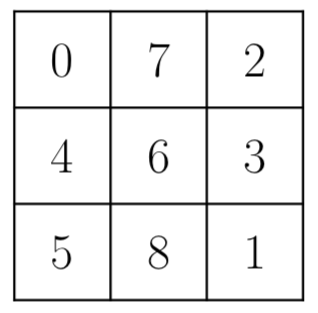There is a $n \times m$ chessboard. Queen’s position right now is $(x,y)$.

Queen is the most powerful chess. It can be moved any number of unoccupied squares in a straight line vertically, horizontally, or diagonally (in all eight directions).

Bad Queen is a greedy queen. It wants to travel around all the blocks on the chessboard, but don’t want to take too many moves to make that happen. Please devise a route such that Bad Queen can visit all the blocks on the chessboard in exactly $nm-1$ moves. The current position is considered as visited.

### 输入格式

The input contains four space-separated integers $n,m,x,y$ ($1 \le x \le n \le 100$, $1 \le y \le m \le 100$, $nm > 1$).

### 输出格式

Output the move sequence in order. That is $nm-1$ lines. Each line contains a coordinate $(x,y)$, separated with space, which is the position after the move.

$x_1 \ y_1 \ x_2 \ y_2 \ \vdots \ x_{nm-1} \ y_{nm-1}$

$(x_i,y_i)$ must be a legal position on chessboard, which means $1 \le x_i \le n$, $1 \le y_i \le m$.

If there are multiple solutions available, you may output any of them.

### 样例

Input
3 3 1 1

Output
3 3
1 3
2 3
2 1
3 1
2 2
1 2
3 2


### 提示

Here is the route in the example. 0 is the starting position, and 1 to 8 are the visiting orders.147 人解决，184 人已尝试。

163 份提交通过，共有 579 份提交。

3.1 EMB 奖励。